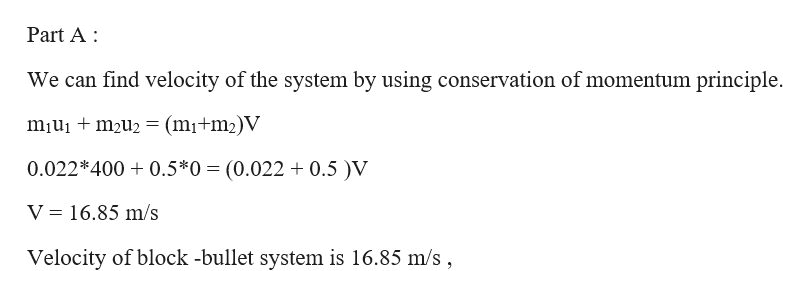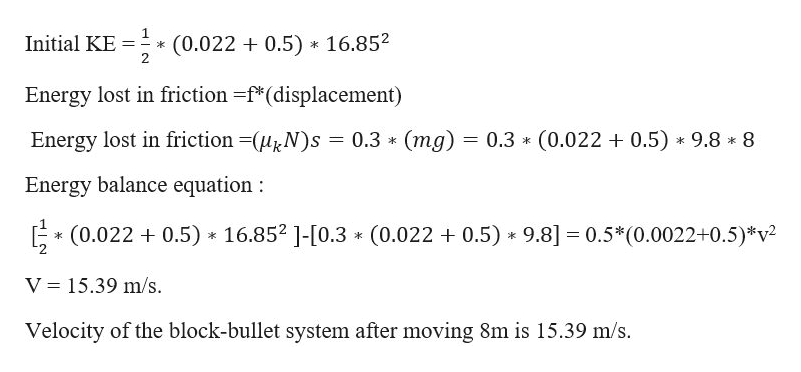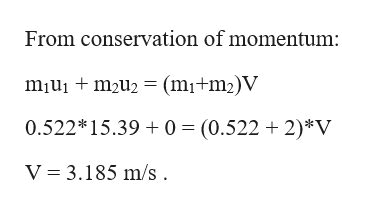A 0.0220 kg bullet moving horizontally at 400 m/s embeds itself into an initially stationary 0.500 kg block.(a) What is their velocity just after the collision?...............m/s(b) The bullet-embedded block slides 8.0 m on a horizontal surface with a 0.30 kinetic coefficient of friction. Now what is its velocity?.................m/s(c) The bullet-embedded block now strikes and sticks to a stationary 2.00 kg block. How far does this combination travel before stopping?................m

Question

A 0.0220 kg bullet moving horizontally at 400 m/s embeds itself into an initially stationary 0.500 kg block.

(a) What is their velocity just after the collision?
...............m/s

(b) The bullet-embedded block slides 8.0 m on a horizontal surface with a 0.30 kinetic coefficient of friction. Now what is its velocity?
.................m/s

(c) The bullet-embedded block now strikes and sticks to a stationary 2.00 kg block. How far does this combination travel before stopping?
................m

Step 1

Given mass of bullet m1 = 0.022 kg

Initial velocity of bullet u1 = 400 m/s

Mass of the block m2 = 0.5 kg

Initial velocity u2 = 0 m/shelp_outlineImage TranscriptionclosePart A We can find velocity of the system by using conservation of momentum principle miuim2u2 (mi+m2)V 0.022*400 0.5*0 (0.022 0.5 )V V 16.85 m/s Velocity of block -bullet system is 16.85 m/s, fullscreen
Step 2

Part b:

We can find velocity of the block by writing the energy balancing equation.

(Initial kinetic energy of the block and bullet system ) -  (work lost against friction )  = (Final kinetic energy of the block and bullet system)help_outlineImage Transcriptionclose1 Initial KE 2 16.85 (0.022 0.5) k Energy lost in friction -f*(displacement) (mg) 0.3 (0.022 0.5) 9.8 8 Energy lost in friction -(HN)s 0.3 * Energy balance equation (0.022 0.5) 16.852 ]-[0.3 (0.022 0.5) 9.8] 0.5*(0.0022+0.5)*v2 k V 15.39 m/s. Velocity of the block-bullet system after moving 8m is 15.39 m/s. fullscreen
Step 3

Part c:

Mass of block-bullet system m1 = 0.022+0.5 = 0.522 kg

Velocity of block-bullet system u1 = 15.39 m/s

Mass of stationa...help_outlineImage TranscriptioncloseFrom conservation of momentum: miuim2(m1+m2)V 0.52215.39 0 (0.522 2)*V V 3.185 m/s. fullscreen

Want to see the full answer?

See Solution

Want to see this answer and more?

Our solutions are written by experts, many with advanced degrees, and available 24/7

See Solution
Tagged in

Physics